# JP5802588B2 - Angle detection device and motor drive control device - Google Patents

## Info

Publication number
JP5802588B2
JP5802588B2 JP2012067256A JP2012067256A JP5802588B2 JP 5802588 B2 JP5802588 B2 JP 5802588B2 JP 2012067256 A JP2012067256 A JP 2012067256A JP 2012067256 A JP2012067256 A JP 2012067256A JP 5802588 B2 JP5802588 B2 JP 5802588B2
Authority
JP
Japan
Prior art keywords
phase
signal
excitation
zero
unit
Prior art date
Legal status (The legal status is an assumption and is not a legal conclusion. Google has not performed a legal analysis and makes no representation as to the accuracy of the status listed.)
Active
Application number
JP2012067256A
Other languages
Japanese (ja)
Other versions
JP2013200141A (en
Inventor

Original Assignee

Priority date (The priority date is an assumption and is not a legal conclusion. Google has not performed a legal analysis and makes no representation as to the accuracy of the date listed.)
Filing date
Publication date
Application filed by 株式会社東芝 filed Critical 株式会社東芝
Priority to JP2012067256A priority Critical patent/JP5802588B2/en
Publication of JP2013200141A publication Critical patent/JP2013200141A/en
Application granted granted Critical
Publication of JP5802588B2 publication Critical patent/JP5802588B2/en
Active legal-status Critical Current
Anticipated expiration legal-status Critical

• 230000005284 excitation Effects 0.000 claims description 138
• 230000000630 rising Effects 0.000 claims description 38
• 238000006243 chemical reactions Methods 0.000 claims description 34
• 238000004364 calculation methods Methods 0.000 claims description 27
• 230000005279 excitation period Effects 0.000 claims description 16
• 230000000875 corresponding Effects 0.000 claims description 13
• 230000001934 delay Effects 0.000 claims description 7
• 230000000051 modifying Effects 0.000 claims description 5
• 238000010586 diagrams Methods 0.000 description 21
• 238000005070 sampling Methods 0.000 description 19
• 241001442055 Vipera berus Species 0.000 description 17
• 230000003111 delayed Effects 0.000 description 13
• 230000004048 modification Effects 0.000 description 7
• 238000006011 modification reactions Methods 0.000 description 7
• 238000003708 edge detection Methods 0.000 description 6
• 241001646071 Prioneris Species 0.000 description 5
• 230000001360 synchronised Effects 0.000 description 4
• 241001330499 Corsiaceae Species 0.000 description 3
• 230000005540 biological transmission Effects 0.000 description 1
• 238000004422 calculation algorithm Methods 0.000 description 1
• 230000004069 differentiation Effects 0.000 description 1
• 238000006073 displacement reactions Methods 0.000 description 1
• 230000000694 effects Effects 0.000 description 1
• 238000000605 extraction Methods 0.000 description 1
• 230000001771 impaired Effects 0.000 description 1
• 238000000034 methods Methods 0.000 description 1
• 230000003252 repetitive Effects 0.000 description 1
• 238000004088 simulation Methods 0.000 description 1
• 230000001960 triggered Effects 0.000 description 1

## Images

•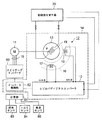•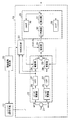•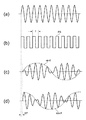•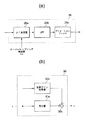•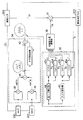•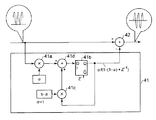•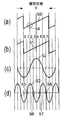•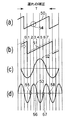•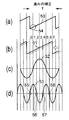••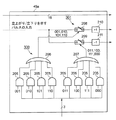•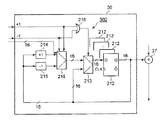•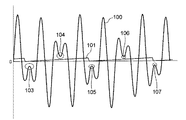•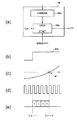•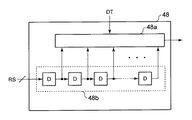## Classifications

• GPHYSICS
• G01MEASURING; TESTING
• G01DMEASURING NOT SPECIALLY ADAPTED FOR A SPECIFIC VARIABLE; ARRANGEMENTS FOR MEASURING TWO OR MORE VARIABLES NOT COVERED IN A SINGLE OTHER SUBCLASS; TARIFF METERING APPARATUS; MEASURING OR TESTING NOT OTHERWISE PROVIDED FOR
• G01D5/00Mechanical means for transferring the output of a sensing member; Means for converting the output of a sensing member to another variable where the form or nature of the sensing member does not constrain the means for converting; Transducers not specially adapted for a specific variable
• G01D5/12Mechanical means for transferring the output of a sensing member; Means for converting the output of a sensing member to another variable where the form or nature of the sensing member does not constrain the means for converting; Transducers not specially adapted for a specific variable using electric or magnetic means
• G01D5/14Mechanical means for transferring the output of a sensing member; Means for converting the output of a sensing member to another variable where the form or nature of the sensing member does not constrain the means for converting; Transducers not specially adapted for a specific variable using electric or magnetic means influencing the magnitude of a current or voltage
• G01D5/20Mechanical means for transferring the output of a sensing member; Means for converting the output of a sensing member to another variable where the form or nature of the sensing member does not constrain the means for converting; Transducers not specially adapted for a specific variable using electric or magnetic means influencing the magnitude of a current or voltage by varying inductance, e.g. by a movable armature
• G01D5/204Mechanical means for transferring the output of a sensing member; Means for converting the output of a sensing member to another variable where the form or nature of the sensing member does not constrain the means for converting; Transducers not specially adapted for a specific variable using electric or magnetic means influencing the magnitude of a current or voltage by varying inductance, e.g. by a movable armature by influencing the mutual induction between two or more coils
• G01D5/2073Mechanical means for transferring the output of a sensing member; Means for converting the output of a sensing member to another variable where the form or nature of the sensing member does not constrain the means for converting; Transducers not specially adapted for a specific variable using electric or magnetic means influencing the magnitude of a current or voltage by varying inductance, e.g. by a movable armature by influencing the mutual induction between two or more coils by movement of a single coil with respect to two or more coils

## Description

One embodiment relates to an angle detection device and a motor drive control device.

The vehicle system applies an excitation wave to the excitation coil of the resolver. The system receives two signal waves from the resolver's sin phase and cos phase coils. The excitation wave has a sinusoidal waveform having a constant amplitude level. The signal wave is an amplitude-modulated wave having a waveform obtained by amplitude-modulating the excitation wave according to the rotation angle. For example, the two-phase signal waves are sin θ · sin ωt and cos θ · sin ωt. ω represents the angular frequency of the excitation wave or excitation signal.

2. Description of the Related Art Conventionally, resolver digital converters are known in which an AC signal output from a resolver is AD (analog to digital) converted to detect a point where the amplitude of the AC signal is zero (see, for example, Patent Documents 1 and 2). There is known a circuit for obtaining a rotation angle by measuring a time difference at a zero cross point at which the voltage level of a two-phase AC signal crosses to zero level (see, for example, Patent Documents 3 and 4).

JP 2011-89885 A US Patent Application Publication No. 2011/0090104 Specification JP 09-37585 A JP 2010-110147 A

However, when the integral multiple of the excitation period and the rotation period of the motor are close to each other, there is a case where the waveform of the signal wave after AD conversion does not pass through the zero level point. As a result, the correction value of the phase is biased, and the error of the angle detection value increases.

As an example, assume that the repetition frequency of the pulse signal of the excitation signal is 10 kHz, and the angular frequency of rotation of the motor is 2.5 kHz. It is assumed that the excitation cycle (1/10 kHz) is four times the motor rotation cycle (1 / 2.5 kHz). The resolver digital converter inputs digital signals sin θ · sin ωt and signals cos θ · sin ωt after AD conversion to the high-pass filter, respectively. The high pass filter distorts each signal. In one period of the signal wave, the amplitude of the signal wave does not reach 0 level at the phase point to be detected as the zero cross point. The resolver digital converter cannot always detect the zero cross point.

In order to solve such a problem, according to one embodiment, an AD conversion unit that performs analog / digital conversion of signal waves of different phases obtained by amplitude-modulating excitation waves with the rotation angle of the rotation axis. And an excitation signal having a reference phase position indicating a reference phase timing within an excitation period and the signal wave of the plurality of phases from the AD converter, and a zero cross phase of a square sum average signal of the signal waves of the plurality of phases Detecting the phase of the excitation signal based on the position of the zero-cross phase and the correction direction of the phase shift at the position of the phase section by equally dividing the excitation cycle, and the phase difference between the phase of the signal wave and the phase of the excitation wave a correction unit for delaying the deviation amount from the reference phase position is, detection of the phase synchronous detection of an output signal from the AD conversion unit in synchronization with the corrected excitation signal If the calculated estimated rotation angle by the output signal of the detector, the an angle calculator for the estimated rotation angle to output to the AD conversion unit, the angle detection apparatus characterized by comprising a are provided.

According to another embodiment, the exciting coil is provided on one of the stator and the rotor of the motor and applied with an exciting wave, and is provided on the other of the rotor and the stator. A detection coil that amplitude-modulates the excitation wave to the coil with a rotation angle of the rotation axis of the rotor to generate a plurality of phase signal waves having different phases, and an AD that performs analog / digital conversion on the plurality of signal waves, respectively. A conversion unit , an excitation signal having a reference phase position indicating a reference phase timing within an excitation cycle, and the plurality of phase signal waves from the AD conversion unit are input, and a zero cross of a square sum average signal of the plurality of phase signal waves detects the phase, the phase section by equal division of the excitation period, based on the correction direction of the position and phase shift of the zero cross phase of the excitation signal phase , The output from the reference phase and a correction unit for delaying displacement amount from the position, the in synchronization with the excitation signal the phase-corrected AD conversion unit is the phase difference between the phase and the signal wave of the phase A detector for synchronously detecting a signal; an angle calculation unit for calculating the rotation angle based on an output signal of the detector; and outputting the calculated rotation angle to the AD conversion unit; and the rotation calculated by the angle calculation unit There is provided a motor drive control device comprising: a control unit that controls an inverter circuit that generates a drive current to the motor according to an angle.

It is a figure which shows the structural example of the vehicle containing the angle detection apparatus and motor drive control apparatus which concern on embodiment. It is a block diagram of the angle detection apparatus which concerns on embodiment. (A)-(d) is a figure which respectively shows the some waveform example of the input and output of a resolver connected to the angle detection apparatus which concerns on embodiment. (A) is a figure which shows the structural example in the AD conversion part of the angle detection apparatus which concerns on embodiment, (b) is a figure which shows the structural example in the angle calculation part of an angle detection apparatus. It is a block diagram of the correction | amendment part of the angle detection apparatus which concerns on embodiment. It is a block diagram of the average value detection part in the square sum average calculation part used for the correction | amendment part of the angle detection apparatus which concerns on embodiment. It is a several time chart for demonstrating the phase correction | amendment of the signal wave of an ideal state in the correction | amendment part of the angle detection apparatus which concerns on embodiment. It is a several time chart for demonstrating the phase correction of the signal wave from which the phase was delayed in the correction | amendment part of the angle detection apparatus which concerns on embodiment. It is a several time chart for demonstrating the phase correction | amendment of the signal wave which the phase advanced in the correction | amendment part of the angle detection apparatus which concerns on embodiment. It is a several figure for demonstrating the rising detection part in the detection part used for the correction | amendment part of the angle detection apparatus which concerns on embodiment. It is a block diagram of the phase correction direction output part in the detection part used for the correction | amendment part of the angle detection apparatus which concerns on embodiment. It is a block diagram of the phase correction amount adjustment part used for the correction | amendment part of the angle detection apparatus which concerns on embodiment. It is a figure which shows the output waveform example of the AD conversion part of the angle detection apparatus which concerns on embodiment. It is a several figure for demonstrating the detection part used for the correction | amendment part of the angle detection apparatus which concerns on the modification of embodiment. It is a block diagram of the phase correction amount adjustment part used for the correction part of the angle detection apparatus which concerns on the modification of embodiment.

Hereinafter, an angle detection device and a motor drive control device according to an embodiment will be described with reference to FIGS. In the drawings, the same portions are denoted by the same reference numerals, and redundant description is omitted.

FIG. 1 is a diagram illustrating a configuration example of a vehicle including an angle detection device and a motor drive control device according to an embodiment.

The resolver digital converter 10 is a digital circuit that detects the rotation angle of the rotating shaft of the motor 11 using a signal from the resolver 12. The rotation angle or rotation angle position refers to the rotation angle of the resolver rotor 13 attached to the rotation shaft of the motor 11.

The motor drive control device 14 includes a cos phase coil 17 and a sin phase coil 18, which are two-phase windings, a resolver digital converter 10, and an inverter control unit 62 (control unit). The motor drive control device 14 obtains a command value that is a drive current value to the motor 11, generates a three-phase current corresponding to the command value based on the resolver output signal, and outputs the current of each phase to the corresponding three lines 15. An LSI (large scale integration) or a processor for supplying the motor 11 to the motor 11.

The motor 11 is a brushless AC motor. The motor 11 is for driving an electric power steering device, for example.

The resolver 12 is a rotation angle sensor of the motor 11. The resolver 12 is provided in the resolver stator 16 fixed to the motor stator, the cos phase coil 17 and the sin phase coil 18 provided in the resolver stator 16, the resolver rotor 13 that rotates together with the motor rotor, and the resolver rotor 13. And an exciting coil 19.

The cos phase coil 17 and the sin phase coil 18 are arranged with a phase difference of 90 degrees from each other. The exciting coil 19 rotates together with the motor rotor and the resolver rotor 13.

The excitation signal generator 20 generates an excitation signal and an excitation pulse signal. The excitation signal is an excitation signal sin ωt having a sinusoidal waveform. The excitation pulse signal is a signal converted from an excitation signal into a pulse signal sequence having the same repetition period as the excitation signal period. sinωt is a time function representing sin (2π / T) · t. Here, π represents the circumference, T represents one cycle of the excitation signal, and t represents time. Further, ωT is represented by deg or rad.

The excitation signal generator 20 includes an exciter that outputs an excitation wave, an amplifier that amplifies the output of the exciter, and a comparator that limits the amplitude level of the amplified excitation wave. The excitation signal generator 20 inputs the excitation signal sin ωt output from the exciter to the excitation coil 19. The excitation signal generator 20 inputs the excitation signal output from the comparator to the resolver digital converter 10.

The motor drive control device 14 includes an inverter control unit 62 that controls the switching inverter 60 (inverter circuit). The switching inverter 60 generates a drive current for the motor 11. The switching inverter 60 includes a plurality of switching elements that generate three-phase voltages to the motor 11. The inverter control unit 62 drives the plurality of switching elements of the switching inverter 60 on and off according to the rotation angle and the command value calculated by the resolver digital converter 10. The vehicle system further includes a calculation unit 61 for obtaining a command value of the motor drive current, a steering angle sensor 63 for steering torque, a torque sensor 64 for steering angle of steering, and a vehicle speed sensor 65 for vehicle speed.

FIG. 2 is a block diagram of the resolver digital converter 10. The above described symbols represent the same elements.

The resolver digital converter 10 receives the two-phase resolver output signal generated by the resolver 12 from the port 21. The two-phase resolver output signal means a sin phase signal sinφ and a cos phase signal cosφ whose phases are different from each other by π / 2. The sin phase signal sinφ is expressed by sinωt · sinθ from the sin phase coil 18. φ represents a time function, and θ represents the rotation angle of the motor 11 estimated by the resolver digital converter 10. The cos phase signal cosφ is expressed as sin ωt · cos θ from the cos phase coil 17.

FIG. 3A to FIG. 3D show a plurality of waveform examples of the input and output of the resolver 12. FIG. 3A shows a waveform of the excitation signal sin ωt. The excitation signal sin ωt is an excitation wave having an excitation period T. FIG. 3B shows the waveform of the excitation signal RS. The excitation signal RS has a repetitive waveform of a plurality of rectangular pulses. The excitation signal RS has an excitation period T. FIG. 3C shows the waveform of the sin phase signal. FIG. 3D shows the waveform of the cos phase signal.

The cos phase signal is obtained by amplitude-modulating the excitation signal sin ωt with the rotation angle θ of the resolver rotor 13 (rotating shaft). The envelope of the amplitude of the cos phase signal changes in a sinusoidal shape with a period longer than the excitation period T.

The sin phase signal is similar to the example of the cos phase signal. The phase of the cos phase signal is delayed with respect to the phase of the excitation signal RS. The phase of the sin phase signal is delayed with respect to the phase of the excitation signal RS. DT represents the phase delay time.

The resolver digital converter 10 in FIG. 2 performs AD conversion on analog sin phase signals and cos phase signals and outputs differential signal waves sin (θ−φ) · sin ωt, and digital sin from the AD conversion unit 22. A phase correction unit 23 (correction unit) that receives the phase signal and the cos phase signal and delays the phase of the excitation signal RS input from the excitation signal generator 20 is provided.

Further, the resolver digital converter 10 includes a detector 24 for synchronously detecting the differential signal waves sin (θ−φ) and sinωt from the AD converter 22, and a rotation angle θ (estimated rotation angle) by the differential signal waves from the detector 24. And an angle calculation unit 25 for calculating. The rotation angle θ refers to estimated θ value data. Detection by the detector 24 is synchronized with the excitation signal RS whose phase is corrected by the phase correction unit 23. The angle calculation unit 25 gives the rotation angle θ to the AD conversion unit 22.

The AD conversion unit 22 includes a first ΔΣ AD converter 26, a first HPF (high pass filter) 27, and a first multiplier 28. The first ΔΣ AD converter 26 converts an analog sin phase signal and outputs a discrete sin phase signal. The first HPF 27 passes the high frequency component of the signal output from the ΔΣ AD converter 26. The first multiplier 28 multiplies the output signal from the HPF 27 and the rotation angle θ input from the angle calculator 25.

The AD conversion unit 22 includes a second ΔΣ AD converter 26, a second HPF 27, and a second multiplier 28 for an analog cos phase signal. Further, the AD conversion unit 22 includes a subtractor 29 that subtracts the output of the second multiplier 28 from the output of the first multiplier 28.

FIG. 4A is a block diagram of the first ΔΣ AD converter 26. The above described symbols represent the same elements.

The ΔΣ AD converter 26 samples an analog signal with an oversampling frequency fos and outputs a pulse density modulation signal, and an LPF (low pass filter) 26b that removes high frequency noise contained in the output of the ΔΣ modulator 26a. And a decimation filter 26c that thins out the output data of the LPF 26b and outputs the obtained 16-bit data at the reference sampling frequency.

The ΔΣ AD converter 26 oversamples the analog signal and generates a digital signal having a necessary resolution. For example, the ΔΣ AD converter 26 generates 16-bit data. The configuration of the second ΔΣ AD converter 26 is the same as that of the first ΔΣ AD converter 26.

The first HPF 27 in FIG. 2 is, for example, a signal differentiation circuit. The first multiplier 28 multiplies sin φ by a cosine function value cos θ of the rotation angle θ. The first multiplier 28 outputs (sin θ · sin ωt) · cos φ.

The second HPF 27 has the same configuration as that of the first HPF 27. The second multiplier 28 multiplies cos φ by a sine function value sin φ of the rotation angle φ. The second multiplier 28 outputs (cos θ · sin ωt) · sin φ.

The subtractor 29 subtracts the output of the second multiplier 28 from the output of the first multiplier 28. The subtractor 29 outputs a differential signal wave sin (θ−φ) · sinωt.

The physical calculation result for the signal by the subtractor 29 is as shown in Expression (I). The difference signal wave sin (θ−φ) · sinωt has a waveform obtained by amplitude-modulating the excitation signal sinωt with the difference signal sin (θ−φ) between the rotation angle φ and the rotation angle θ.

(Sinθ · sinωt) · cosφ− (cosθ · sinωt) · sinφ
= (Sinθ · cosφ−cosθ · sinφ) · sinωt
= Sin (θ−φ) · sinωt (I)
2 delays the phase of the excitation signal RS. The amount of delay by the phase correction unit 23 is DT (see FIG. 3D). The delay amount DT is a phase lag (phase difference) between the phase of the two-phase signal wave and the excitation signal phase.

FIG. 5 is a block diagram of the phase correction unit 23. The above described symbols represent the same elements.

The phase correction unit 23 adds the square of the cos phase signal and the square of the sin phase signal, calculates the time average value of the addition result, and outputs a square sum average signal having a half period of the excitation period T. The square sum average calculator 34 and the zero point detector 35 (detection) for detecting the phase of the square sum average signal at the timing when the amplitude of the square sum average signal is 0 (reference level) and obtaining the phase shift amount Part).

The phase shift amount is a phase shift amount between the phase at the zero cross point of the ideal signal waveform and the phase at the zero cross point of the actual input signal waveform. The amount of phase shift is represented by a value within one excitation cycle T of the square sum average signal. The value is in the range of -90 deg to +90 deg. The phase correction unit 23 outputs the square sum average signal in 16 bits.

The phase correction unit 23 further includes a phase correction amount adjustment unit 36 that corrects the phase of the square sum average signal based on the phase shift amount obtained by the zero point detection unit 35, and ports 201, 202, 203, and 204. Yes. The phase correction unit 23 includes an adder 37 that adds the phase correction amount information 0, +1, or −1 from the phase correction amount adjustment unit 36 to the 16-bit excitation signal RS input from the port 202.

The sum-of-squares calculation unit 34 includes a multiplier 38 that squares the sin phase signal (sin θ · sin ωt) from the port 200, and a multiplier 39 that squares the cos phase signal (cos θ · sin ωt) from the port 201; And an adder 40 for adding the output of the multiplier 38 and the output of the multiplier 39.

The output of the adder 40 is represented by 16 bits. Its value in decimal is always equal to or greater than 0 (see the output waveform of adder 40 in FIG. 5). The physical calculation result on the signal by the square sum average calculation unit 34 is as shown in Expression (II).

(Sin θ · sin ωt) 2 + (cos θ · sin ωt) 2
= (Sin 2 θ + cos 2 θ) · sin 2 ωt
= Sin 2 ωt = (1−cos 2ωt) / 2 (II)
Further, the square sum average calculation unit 34 includes an average value detection unit 41 that calculates a time average value of the output of the adder 40, and a subtractor 42 that subtracts the output of the average value detection unit 41 from the output of the adder 40. Yes.

FIG. 6 is a block diagram of the average value detection unit 41. The above described symbols represent the same elements. The average value detector 41 calculates a time average of values represented by input 16-bit data. The average value detection unit 41 includes a 16-bit multiplier 41a that multiplies the nth 16-bit data by the parameter α, and a 16-bit flip-flop 41b (the figure shows only one bit). ). n represents a natural number and corresponds to, for example, a sampling time.

The average value detector 41 multiplies the (n−1) -th 16-bit data from the flip-flop 41b by a parameter (1-α), and adds the output of the multiplier 41a and the output of the multiplier 41c. And an adder 41d. The parameters α and α-1 are both in the range of 0 to 1.

The processing by the average value detection unit 41 is subtraction by subtracting a determined percentage amount of the (n-1) th 16-bit data before one sampling from the nth 16-bit data. When the number of samplings increases, the average value detection unit 41 converges the input 16-bit data to the average value.

The square sum average calculator 34 adds the square of the sin phase signal and the square of the cos phase signal, and subtracts the time average value of the addition result from the addition result. The square sum average calculator 34 outputs a square sum average signal that vibrates at twice the frequency of the original sin phase signal (see the output waveform of the square sum average calculator 34 in FIG. 5).

After the initial value of the 16-bit data is given, the average value detection unit 41 continues to always add the average value of the amplitude of the square sum average signal. Or the average value detection part 41 resets the average value of the amplitude of a square sum average signal for every predetermined time interval. The average value detection unit 41 may repeat resetting and resuming addition.

5 detects the timing at which the amplitude of the square sum average signal zero-crosses. The square sum average signal has four zero cross points within one excitation period T.

FIG. 7D shows a waveform example of the square sum average signal in the ideal state. The square sum average signal 53 has zero cross points 55, 56, 57 and 58. The zero point detection unit 35 uses four phases of zero cross points 55 to 58 of the square sum average signal 53 in the ideal state as reference phases. In the ideal state, the amount of phase shift is zero.

FIG. 8D shows a square sum average signal 53 having a phase delayed from the reference phase of FIG. FIG. 9D shows a square sum average signal 53 having a phase advanced from the reference phase of FIG.

In FIG. 7D, the first zero cross point 55 of the square sum average signal 53 is located on the boundary between the phase sections 0 and 1. In FIG. 8D, the zero cross point 55 is located on the boundary between the phase sections 1 and 2. In FIG. 9D, the zero cross point 55 is located on the boundary between the phase sections 7 and 0.

As shown in FIG. 8D, the zero cross point 55 that should originally appear on the boundary between the phase sections 0 and 1 appears at a position delayed by one section width. As shown in FIG. 9D, the zero cross point 55 that should originally appear on the boundary between the phase sections 0 and 1 appears at a position advanced by one section width.

The processing by the zero point detection unit 35 detects the phase of the square sum average signal 53 at the zero cross point 55. The zero point detection unit 35 obtains a phase shift amount from a reference position (a reference phase position of the phase within the excitation cycle T) such as on the boundary between the phase sections 0 and 1, for example, as a known excitation wave signal 50 (excitation signal). It is obtained from the reference phase timing.

The zero point detector 35 determines whether the detected phase is delayed or advanced from the boundary between the phase sections 0 and 1 for the input signal waveform. Based on the determination result, the zero point detection unit 35 generates correction direction information indicating a direction for advancing the delayed phase or a direction for returning the advanced phase, and correction amount information.

The processing of the zero point detection unit 35 for the zero cross points 56, 57 and 58 is substantially the same as the example of the zero cross point 55.

The configuration of the zero point detection unit 35 will be described. Returning to FIG. 5, the 0 point detector 35 detects the rising timing at which the signal waveform crosses to 0 level while the signal waveform rises, and the falling timing at which the signal waveform crosses to 0 level while the signal waveform falls. And a falling edge detection unit 44 for detection. The signal waveform refers to the waveform of the square sum average signal 53.

FIG. 10A is a diagram illustrating a configuration example of the rising edge detection unit 43. For example, a comparator 59 is used for the rise detection unit 43. The comparator 59 has, for example, two input terminals. One input terminal is grounded to a potential of zero.

FIG. 10B is a diagram illustrating a waveform of the square sum average signal 53 input to the comparator 59. The comparator 59 receives the square sum average signal 53 from the other input terminal.

FIG. 10C is a diagram illustrating an example of an output signal from the comparator 59. The timing of zero cross detection by the rising edge detection unit 43 corresponds to a positive edge clock of a signal from Low to High.

The configuration of the falling detection unit 44 is substantially the same as the configuration of the rising detection unit 43. The timing of zero cross detection by the falling detection unit 44 corresponds to a negative edge clock of a signal from High to Low. The start timing of the operation of the falling detection unit 44 is different from the start timing of the operation of the rising detection unit 43.

In FIG. 5, the zero point detection unit 35 includes two phase correction direction output units 45 a and 45 b on the output side of the rise detection unit 43, and two phase correction direction output units 45 c and 45 d on the output side of the fall detection unit 44. (Numbers 1, 2, 5, 6 and 3, 4, 7, 0 will be described later).

FIG. 11 is a block diagram of the phase correction direction output unit 45a. The above described symbols represent the same elements.

The phase correction direction output unit 45a indicates the section numbers (numbers) 0, 1, 2, 3, 4, 5, 6, 7 of the phase sections by dividing the excitation period T into eight equal parts, and the positions of the zero cross phases in the ideal state. The correction direction of the phase shift is output according to the reference phase position to be expressed.

The correction direction means a positive direction for advancing the phase and a negative direction for returning the phase. Section numbers 0 to 7 are eight phase sections with order obtained by dividing the excitation cycle T. Section numbers 0 to 7 indicate which of the eight phase sections the zero-cross phase of the square sum average signal at the rising timing belongs to.

The phase correction direction output unit 45a includes a distribution unit 300 that determines the section number of the zero-crossing phase of the rising timing based on the digital amplitude level of the digital excitation signal 51, a rising or falling detection pulse, section numbers 0 to 7 and a reference And an output unit 301 that outputs a correction direction and a correction amount of the phase shift according to the phase position. The excitation signal 51 (FIG. 7B) after AD conversion is represented by 16 bits.

When an arbitrary zero cross occurs, the phase correction direction output unit 45a indicates that the phase at the zero cross point of the square sum average signal is on the boundary of any one of phase sections 0 to 7 or adjacent phase sections. Find what is located at. After obtaining the phase position, the phase correction direction output unit 45a obtains the phase correction direction and the correction amount.

FIG. 7A is a time chart of the excitation sawtooth wave in which each phase is located at the reference phase position. The excitation sawtooth signal 50 in the figure corresponds to the output of the port 202 in FIG. It is assumed that the excitation period T is (1/10 kHz) seconds. The excitation sawtooth signal 50 is an original waveform for extraction processing of the amount of phase shift.

FIG. 7B is a time chart of the excitation sawtooth wave after the phase is adjusted in the ideal state. The excitation sawtooth wave 51 in the figure corresponds to the output of the port 203. FIG. 7C is a time chart of a sin phase signal after AD conversion by the AD converter 22 in an ideal state. The figure shows a sin phase signal at the port 200.

FIG. 7D is a time chart of the square sum average signal in the ideal state. The frequency of the square sum average signal 53 is twice the frequency of the sin phase signal 52. In FIG. 7A to FIG. 7D, each time chart shares a time axis.

The excitation sawtooth signal 50 takes a value in the range from the bottom value 0x0000 to the top value 0xffff. 0x represents a hexadecimal number. The value of the excitation sawtooth signal 50 is 0x4000 at point 54.

FIG. 8A to FIG. 8D show the relationship between the waveform and the phase when correcting the phase of the square sum average signal 53 whose phase is delayed.

FIG. 8A is a time chart of the excitation sawing signal 50 whose phase is delayed. FIG. 8B is a time chart of the excitation sawtooth wave 51 in the phase delay state. FIG. 8C is a time chart of the sin phase signal 52 in the phase delay state. FIG. 8D is a time chart of the square sum average signal 53 in the phase delay state.

FIG. 9A to FIG. 9D show the relationship between the waveform and the phase when correcting the phase of the square sum average signal 53 with the phase advanced.

FIG. 9A is a time chart of the excitation sawing signal 50 whose phase has advanced. FIG. 9B is a time chart of the excitation sawtooth wave 51 in the phase advance state. FIG. 9C is a time chart of the sin phase signal 52 in the phase advance state. FIG. 9D is a time chart of the square sum average signal 53 in the phase advance state.

The same reference numerals in FIG. 7A to FIG. 9D denote elements that are substantially equivalent to each other.

The phase correction direction output unit 45a obtains the phase of the signal waveform at the time of zero crossing of the square sum average signal 53 using the upper 3 bits of the 16-bit data fed back from the adder 37.

When the first rising zero cross point 55 is located on the boundary between the phase sections 1 and 2 within the period T as shown in FIG. 8D, the phase correction direction output unit 45a outputs the excitation signal 51 after AD conversion. Advance the phase. The amount by which the 16-bit phase data is advanced by the phase correction direction output unit 45a is one sampling rate. One sampling rate refers to a sampling time width sufficiently shorter than a time length corresponding to one section width. Data within one sampling time is shifted by one sampling rate. By repeating the shift a plurality of times, the phase correction direction output unit 45a moves the data of the excitation signal 51 to the right by one section as shown in FIG.

When the first rising zero cross point 55 within the period T is located on the boundary between the phase sections 7 and 0 as shown in FIG. 9D, the phase correction direction output unit 45a outputs the excitation signal 51 after AD conversion. The phase is delayed by one interval. The phase correction direction output unit 45a delays the 16-bit phase data by one sampling rate. By repeating the shift a plurality of times, the phase correction direction output unit 45a moves the data of the excitation signal 51 one section to the left as shown in FIG. 9B.

The maximum amount of phase shift that can be corrected by the phase correction direction output unit 45a is an amount corresponding to two section widths. The two section widths correspond to the phase 90 deg of the square sum average signal 53. The phase correction direction output unit 45a corrects the delay of the two section widths or the advance of the two section widths in the signal waveform of FIG. Even if the zero cross point 55 on the boundary between the phase intervals 0 and 1 in FIG. 7D is shifted to just before the end in the phase interval 2 in the same figure, the phase correction direction output unit 45a corrects the shift amount. To do.

The distribution unit 300 shown in FIG. 11 includes eight AND gates 205 and OR gates 206 and 207. Three bits common to the AND gates 205 are input to the eight AND gates 205, respectively. 3 bits means the upper 3 bits of 16 bits. 16 bits represent the range of 32767 to -32768 which the amplitude of the excitation signal 51 after AD conversion takes.

Eight AND gates 205 are input with different 3-bit pattern data. The phase correction direction output unit 45a stores the 3-bit pattern data in advance. The output unit 301 outputs a switch 208 that closes the circuit when the detection pulse information and the OR gate 206 are turned on, a switch 209 that closes the circuit when the detection pulse information and the OR gate 207 is turned on, and a signal representing “plus 1”. 210 and a calculator 211 that outputs a signal representing “minus 1”.

The phase correction direction output units 45b, 45c and 45d have substantially the same configuration as that of the phase correction direction output unit 45a.

Among the phase correction direction output units 45 a to 45 d, the phase correction direction output units 45 a and 45 b are notified of the rising detection pulse from the rising detection unit 43. The phase correction direction command units 45 c and 45 d are notified of the falling detection pulse from the falling detection unit 44.

The first phase correction direction output unit 45a is for correcting the phase of the square sum average signal 53 in which the detection phase at the rising zero cross point appears in the phase sections 1 and 2 (see FIG. 8D). is there. As shown in the figure, the square sum average signal 53 has two detection points rising within one excitation period T (zero cross points 55 and 57). The phase correction direction output unit 45 a detects both the phase shift of the zero cross point 55 in the first half phase sections 1 and 2 and the phase shift of the zero cross point 57 in the second phase sections 5 and 6. Correction is performed by correcting the phase shift amount.

The second phase correction direction output unit 45b is for correcting the phase of the square sum average signal 53 in which the detection phase at the rising zero cross point appears in the phase sections 7 and 0 (see FIG. 9D). is there. As shown in the figure, the square sum average signal 53 has two detection points rising within one excitation period T (zero cross points 55 and 57). The phase correction direction output unit 45b detects both the phase shift of the zero cross point 55 in the first half phase section 7 and 0 and the phase shift of the zero cross point 57 in the second phase section 3 and 4, and the phase shift direction of the first zero cross point 55. Correction is performed by correcting the phase shift amount.

Similarly, the third phase correction direction output unit 45d corrects the phase of the square sum average signal 53 in which the detection phase at the falling zero-cross point appears in the phase sections 3 and 4 (see FIG. 8D). Is for. As shown in the figure, the square sum average signal 53 has two detection points falling within one excitation cycle T (zero cross points 56 and 58). The phase correction direction output unit 45d outputs both the phase shift of the zero cross point 56 in the first half phase section 3 and 4 and the phase shift of the zero cross point 58 in the second phase section 7 and 0 to the phase shift of the zero cross point 56 of the first half. Correction is performed by correcting the phase shift amount.

The fourth phase correction direction output unit 45c is for correcting the phase of the square sum average signal 53 in which the detection phase at the falling zero cross point appears in the phase sections 1 and 2 (see FIG. 9D). It is. As shown in the figure, the square sum average signal 53 has two detection points rising within one excitation cycle T (zero cross points 56 and 58). The phase correction direction output unit 45 c detects both the phase shift of the zero cross point 56 in the first half phase sections 1 and 2 and the phase shift of the zero cross point 58 in the second phase sections 5 and 6. Correction is performed by correcting the phase shift amount.

The phase correction direction output units 45a, 45b, 45c, and 45d are commands that indicate a phase correction direction according to a detection pulse (detection information) for identifying rising or falling and a phase position of a rising or falling zero-cross point. To the phase correction amount adjusting unit 36.

FIG. 12 is a block diagram of the phase correction amount adjustment unit 36. The above described symbols represent the same elements. The phase correction amount adjustment unit 36 includes 16 flip-flops 212 (delay elements) corresponding to 16 bits representing the amplitude level of the excitation signal RS, an input-side selection unit 302, and an arithmetic circuit on the preceding stage of each flip-flop 212. 213. The selection unit 302 switches whether to advance or return the phase of the square sum average signal 53 or pass the square sum average signal 53 according to the phase shift correction direction and correction amount. The selection unit 302 includes an OR gate 216, an arithmetic unit 214 that outputs a signal representing “plus 1”, an arithmetic unit 215 that outputs a signal representing “minus 1”, and an arithmetic circuit 216. The arithmetic circuit 213 performs holding of the value of the 16-bit flip-flop 212, incrementing of each value, or decrementing of each value by selection by the selection unit 302.

Returning to FIG. 2, the angle calculation unit 25 integrates the angular velocity from the angular velocity calculation unit (PI [Proportion Integration] controller) 30 that calculates the angular velocity of the motor 11 and the angular velocity calculation unit 30 to obtain the rotation angle θ of the motor 11. A rotation angle calculation unit (integrator) 31 to be calculated, a COS table 32 that outputs a cosine function value cos θ corresponding to the rotation angle θ from the rotation angle calculation unit 31 to the first multiplication unit 28, and a rotation angle θ And a SIN table 33 for outputting the sine function value sin θ to the second multiplier 28.

FIG. 4B is a block diagram of the angular velocity calculation unit 30. The angular velocity calculation unit 30 integrates the control deviation ε from the detector 24 to calculate the angular velocity of the motor 11, and a proportional gain adjuster for inserting a feedback gain of a proportional element into the output of the integrator 30a. 30b and an adder 30c for adding the output of the proportional gain adjuster 30b to the output of the integrator 30a. The calculation by the angular velocity calculation unit 30 is PI (proportional + integral) control using adjustment of the proportional gain and phase compensation by the integrator 30a.

The vehicle system having the resolver digital converter 10 (FIG. 1) having such a configuration collects information from the torque sensor 64 and the like by the calculation unit 61. The inverter control unit 62 generates a three-phase PWM (pulse width modulation) control voltage signal having a pulse width corresponding to the target voltage input to the motor 11, and outputs each voltage signal to the switching inverter 60. The switching inverter 60 generates a three-phase AC drive current corresponding to each PWM control voltage signal and supplies the generated current to the motor 11. A three-phase AC drive current is a sinusoidal current. The motor rotation shaft rotates.

The system causes the excitation signal generator 20 to apply an excitation pulse signal to the resolver digital converter 10. The excitation signal generator 20 applies an excitation signal to the resolver 12. As the resolver rotor 13 rotates, the excitation coil 19 rotates. The positional relationship between the resolver rotor 13 and the resolver stator 16 is changed by the shaft rotation. A magnetic field is induced. The resolver 12 causes a phase delay of the excitation signal by its operation. Signal transmission between the resolver 12 and the resolver digital converter 10 causes a phase delay.

The two ΔΣ AD converters 26 of the AD conversion unit 22 oversample the sin phase signal and the cos phase signal. The number of bits of the output of the ΔΣ AD converter 26 depends on the decimation ratio. The decimation ratio is the ratio between the oversampling rate of the decimation filter 26c and a predetermined sampling rate. The decimation ratio is 16. As a result, the two HPFs 27 distort the waveform of the output signal of each ΔΣ AD converter 26.

FIG. 13 is a diagram illustrating an output waveform example of the AD conversion unit 22. This figure shows the results obtained by the inventors through simulation. A waveform 100 in the figure shows a digital sin phase signal or a digital cos phase signal. The horizontal axis represents time, and the vertical axis represents deg. A waveform 101 represents a motor rotation angle. The condition is that the rotation frequency of the motor 11 is 2500 Hz and the frequency of the excitation signal is 10 kHz. As for the shape of the waveform 100, the waveform 100 has a substantially left-right symmetry with respect to a vertical line passing through the point 105. Waveform 100 has points 103, 104, 105, 106, and 107 that do not cross zero, respectively. The two HPFs 27 distort the waveform.

The processing by the resolver digital converter 10 corrects the phase of the square sum average signal so that the waveform 100 zero-crosses at the zero-cross point where the waveform 100 should originally pass.

From the principle of operation of the resolver 12, the signal from the AD converter 22 is expressed by the formula (III).

SIN phase side K · ext (t) · sin (φ)
COS phase side K · ext (t) · cos (φ) (III)
Here, K represents a coefficient of a route on the way. ext (t) represents an excitation signal. φ represents the rotation angle of the motor shaft. When the sum of squares of each is calculated, the square of the excitation signal is obtained as shown in equation (IV).

[K · ext (t) · sin (φ)] 2 + [K · ext (t) · cos (φ)] 2 = K 2 · ext (t) 2 (IV)
The resolver digital converter 10 subtracts the average value from the signal represented by the formula (IV). The resolver digital converter 10 adjusts the phase by matching the zero-cross point of the signal obtained by subtraction and the phase of excitation as described in detail below.

In FIG. 5, the phase correction unit 23 receives the excitation signal RS from the port 202. The phase correction unit 23 receives sin phase signals and cos phase signals from the ports 201 and 202. The zero point detection unit 35 receives the square sum average signal from the phase correction unit 23. The zero point detector 35 receives the upper 3 bits of the 16-bit amplitude data of the excitation signal 51 after AD conversion from the adder 37.

Unlike the ideal state, the zero point detector 35 receives a square sum average signal having a phase shift. In the square sum average signal input to the zero point detection unit 35, the rising zero cross is detected by the rising detection unit 43 first. After the start of the operation of the rise detection unit 43, the operation of the fall detection unit 44 starts. The square sum average signal is first detected for a rising zero cross by the rising detection unit 43, and then detected for a falling zero cross by the falling detection unit 44.

The rising edge detection unit 43 detects an unknown rising zero cross point. The rising detection unit 43 notifies the phase correction direction command units 45a and 45b of a detection pulse indicating that the rising zero cross has been detected. The phase correction direction output units 45a and 45b refer to the same upper 3 bit data triggered by the input of the detection pulse. The phase correction direction output units 45a and 45b extract whether the phase position of the zero cross point is located in the phase interval 0 to 7 or in the boundary between the intervals.

If the 3-bit pattern is any one of 001, 010, 101, and 110, the phase correction direction output unit 45a has a zero cross point in any one of the section numbers 1, 2, 5, and 6, and determines the delay. To do. The switch 208 closes the circuit by the rising information indicated by the detection pulse and when the OR gate 206 is turned on. The phase correction direction output unit 45a outputs a command for advancing the phase together with the 16-bit square sum average signal. When the first zero cross point is detected, the phase correction direction output unit 45a outputs a command to advance the phase together with the square sum average signal at the detection timing of the second zero cross point.

If the 3-bit pattern is any one of 011, 100, 111,000, the phase correction direction output unit 45b indicates that the zero cross point is in any one of the section numbers 3, 4, 7, and 0. Is determined. The switch 209 closes the circuit by the rising information indicated by the detection pulse and the OR gate 207 being turned on. The phase correction direction output unit 45a outputs a command for delaying the phase together with a 16-bit square sum average signal. When the first zero cross point is detected, the phase correction direction output unit 45b outputs a command for delaying the phase together with the square sum average signal at the detection timing of the second zero cross point.

Similarly to the rising example, when the falling detection unit 44 detects an unknown zero-cross point of the falling, it notifies the phase correction direction command units 45c and 45d of a detection pulse indicating the falling. The phase correction direction output units 45c and 45d refer to the upper 3 bit data.

If the 3-bit pattern is any one of 001, 010, 101, and 110, the phase correction direction output unit 45c has the zero cross point in any one of the section numbers 1, 2, 5, and 6, and determines the advance. To do. The phase correction direction output unit 45c outputs a command for delaying the phase together with the square sum average signal. When the first zero cross point is detected, the phase correction direction output unit 45c outputs a command for delaying the phase together with the square sum average signal at the detection timing of the second zero cross point.

If the 3-bit pattern is any one of 011, 100, 111,000, the phase correction direction output unit 45d has a zero cross point in any one of the section numbers 3, 4, 7, 0, and is delayed. Is determined. The phase correction direction output unit 45d outputs a command for advancing the phase together with the square sum average signal. When the first zero cross point is detected, the phase correction direction output unit 45d outputs a command for advancing the phase together with the square sum average signal at the detection timing of the second zero cross point.

The 16-bit data by the phase correction direction command units 45a to 45d is shifted at a high sampling time such as 4 μsec. The zero point detector 35 operates at a sampling rate having a time sufficiently shorter than the time width of one phase interval. The phase correction direction command units 45a to 45d output a command signal for advancing or delaying the phase at the sampling rate.

The phase correction amount adjusting unit 36 performs a process of +1 or −1 on 16-bit data corresponding to one section width at a sampling interval. The 16-bit data output by the phase correction amount adjustment unit 36 at the sampling rate moves to the adder 37 at the same sampling rate. The 16-bit data from the adder 37 is input to the zero point detector 35 at the sampling rate. The phase correction amount adjustment unit 36 repeats corrections of +1 and −1 for each sampling interval.

The zero point detection unit 35, the phase correction amount adjustment unit 36, and the adder 37 continue the processing in the loop formed by them by sampling a plurality of times. As a result, phase correction corresponding to the time length of one phase interval is completed. The phase amount for up to two phase intervals is delayed or advanced. Temporarily correcting an amount of phase corresponding to the time length of one phase interval causes an unexpected jump due to the influence of noise. The phase correction amount adjusting unit 36 executes the loop processing a plurality of times and shifts the phase by a small amount, whereby the phase of the square sum average signal approaches the phase of the ideal state.

When rising or falling is detected in this manner, the phase is corrected by the section number of the detected phase. The phase of the excitation signal at the extracted zero cross point is detected, and the correction direction of the square sum average signal is obtained according to the detected phase. By executing the loop processing repeatedly, the correction amount of the excitation signal having the phase of the deviation amount in the range of 90 degrees to -90 degrees is also appropriately corrected. The phase shift of the excitation signal is adjusted.

In the conventional example, when an integral multiple of the excitation period T and the rotation period of the motor 11 are close to each other, there is a technical problem that the waveform after AD conversion does not necessarily pass through the “0” point. As a result, the correction value is biased, and as a result, the error of the detected angle value may increase. According to the resolver digital converter 10 and the motor drive control device 14, by adjusting the phase of the binary sum of the input signal and the phase of the excitation signal, the error of the angle detection value is eliminated and the technical problem is overcome. The

For example, if the excitation period (1/10 kHz) is four times the rotation period (1 / 2.5 kHz) of the motor 11, the digital sin phase signal and the digital cos phase signal are distorted by the HPF 27 as shown in FIG. The phase correction using the digital sin phase signal and the digital cos phase signal has low accuracy. According to the angle detection device and the motor drive control device according to the embodiment, since the digital sin phase signal and the digital cos phase signal are squared and the zero cross point of the square sum average signal is used, the accuracy is improved.

(Modification)
The angle detection device and the motor drive control device according to the embodiment may use another zero cross point detection unit instead of the zero point detection unit 35. The angle detection device and the motor drive control device according to the modification have substantially the same configurations as the resolver digital converter 10 and the motor drive control device 14, respectively, unless otherwise specified.

FIG. 14A is a block diagram of a detection unit used in an angle detection device according to a modification. The above described symbols represent the same elements.

The phase delay time measurement unit 46 (detection unit) measures the phase delay time DT. The phase delay time measurement unit 46 detects the phase of the square sum average signal at the zero cross timing, and obtains the phase shift amount.

As shown in FIGS. 5 and 14A, the phase delay time measurement unit 46 detects another zero point detection unit that detects the zero-cross point of the square sum average signal from the output of the adder 40 (FIG. 5). 46a and a counter 46b to which the detection signal from the zero point detection unit 46a and the excitation signal RS from the port 202 (FIG. 5) are input. The phase delay time measurement unit 46 starts counting by the counter 46b from the rising timing of the excitation signal RS. The phase delay time measurement unit 46 causes the counter 46b to stop counting by the detection signal of the rising timing or the detection signal of the falling timing.

FIG. 14B is a time chart of the excitation signal RS. FIG. 14C is a time chart of the square sum average signal waveform. FIG. 14D is a time chart of the clock of the operation of the counter 46b. FIG. 14E is a time chart of the value of the counter 46b. The above described symbols represent the same elements.

It is assumed that the initial value of the counter 46b is 0x0000. The counter 46b starts counting when the excitation signal RS rises. The counter 46b continues to increase in value with the input of the clock. The counter 46b is notified by the detection signal from the zero point detector 46b that the amplitude level represented by the 16-bit digital data has become zero. The counter 46b stops counting. The phase delay time measurement unit 46 outputs the count value 4 as DT.

The phase delay time measuring unit 46 directly detects the zero cross point 47 of the square sum average signal using the excitation signal RS as an original signal. The phase delay time measurement unit 46 can detect the phase shift amount with high accuracy.

Further, another phase correction amount adjustment unit instead of the phase correction amount adjustment unit 36 may be used in the angle detection device and the motor drive control device according to the embodiment.

FIG. 15 is a block diagram of a phase correction amount adjustment unit used in an angle detection device according to a modification. The above described symbols represent the same elements.

The phase correction amount adjusting unit 48 delays the excitation signal RS by a phase delay (phase difference) corresponding to the phase delay time DT obtained by the phase delay time measuring unit 46, and the delayed excitation signal RS is detected by the detector 24 (FIG. 2). Output to.

The phase correction amount adjusting unit 48 includes a register circuit (multi-stage delay unit) 48b having 16-bit flip-flops D connected in series to each other in series, and a plurality of flip-flops D according to section numbers 0 to 7 And a switching circuit (tap switching unit) 48a for selecting one or a plurality of flip-flops D. The 16 flip-flops D are flip-flops D representing serial 16-bit excitation signals obtained by parallel-to-serial conversion of 16-bit excitation signals.

The register circuit 48b receives the excitation signal RS from the leading flip-flop D. The register circuit 48b sequentially delays the excitation signal RS. The register circuit 48b has a plurality of taps. A tap means the output of each flip-flop D.

The switching circuit 48a switches and selects a plurality of taps of the register circuit 48b according to the magnitude of the phase delay time DT from the phase delay time measuring unit 46, and generates a synchronization signal. The switching circuit 48a outputs the corrected excitation signal from the selected flip-flop D.

By the phase delay time DT, the phase shift amount adjustment unit 48 is notified of the phase shift amount of the excitation signal RS. The required number of delay elements among the plurality of delay elements is selected by the phase delay time DT. As a result, the number of flip-flops D selected represents the phase correction amount. The phase shift amount of the excitation signal is corrected. The corrected excitation signal for synchronous detection is input to the detector 24.

Comparing the phase correction amount adjusting unit 36 and the phase correction amount adjusting unit 48 of FIG. 12, the phase correction amount adjusting unit 36 does not connect the delay elements in series, and the phase in which the delay elements are connected in series. Different from the correction amount adjustment unit 48. The circuit of the phase correction amount adjustment unit 36 is simpler than the circuit of the phase correction amount adjustment unit 48. The circuit scale of the phase correction amount adjustment unit 36 without the connection of the serial delay element is smaller than the circuit scale of the phase correction amount adjustment unit 48 with the connection.

(Other)
Each block diagram is exemplary.

Although the above embodiment discloses the best mode, the resolver digital converter 10 may detect the zero cross point using only one of the rising edge detection unit 43 and the falling edge detection unit 44. The resolver digital converter 10 may detect only the rising cross points 55 and 57 or only the falling cross points 56 and 58.

The accuracy of the phase detection by the resolver digital converter 10 having both the rising detection unit 43 and the falling detection unit 44 is higher than that by the resolver digital converter 10 having only one of them.

In the above description, the zero cross point uses the voltage value 0 as the amplitude level of the square sum waveform, but a predetermined voltage value may be used instead of the zero cross point. The superiority of the angle detection device according to the present embodiment with respect to the product implemented using voltage values other than the zero cross point is not impaired.

In the above description, the cos φ signal and the sin φ signal have two phases, but the angle detection device according to the embodiment may use signal waves of three phases or four phases or more. The amplitudes of sin φ and cos φ are 1, but it goes without saying that the amplitude may be different from 1.

In the above description, the excitation coil 19 is rotating and the sin phase coil 17 and the cos phase coil 18 are fixed. The side that induces the magnetic field and the side that induces the magnetic field may be disposed relatively opposite to each other. The angle detection device and the motor drive control device according to the embodiment may use an arrangement in which the excitation coil 19 is fixed and the sin phase coil 17 and the cos phase coil 18 are rotated.

In the above description, the ratio between the excitation period (1/10 kHz) and the rotation period (1 / 2.5 kHz) of the motor 11 is 1: 4. The relationship between the phase of the excitation signal and the phase of the rotation angle of the motor 11 is not limited to 1: 4 at the timing when the amplitude of the signal wave output from the ΔΣ AD converter 26 becomes 0 level. For example, using integers j and k, when j times the excitation cycle and k times the rotation cycle of the motor 11 coincide, the excitation signal crosses zero, and the rotation angle of the motor 11 crosses zero. Even in the case of this pair relationship of integers j and k, the angle detection device and the motor drive control device according to the embodiment can achieve the same effects as the above example.

The function of the resolver digital converter 10 is mainly realized by ASIC (application specific integrated circuit) or FPGA (field-programmable gate array). An analog circuit is used for the signal input port in the resolver digital converter 10 and the AD converter 22. The algorithm operation in the above description is a physical operation on a signal using a gate element.

In the above description, the AD conversion unit 22 outputs the differential signal wave sin (θ−φ) · sinωt, and the detector 24 multiplies the differential signal wave sin (θ−φ) · sinωt by the excitation signal RS to obtain the differential signal. sin (θ−φ) was output. The resolver digital converter 10 may perform synchronous detection on the output signal of the HPF 27 in the AD converter 22.

Although several embodiments have been described, these embodiments have been presented by way of example and are not intended to limit the scope of the invention. These embodiments can be implemented in various other forms, and various omissions, replacements, and changes can be made without departing from the spirit of the invention. These embodiments and their modifications are included in the scope and gist of the invention, and are also included in the invention described in the claims and the equivalents thereof.

DESCRIPTION OF SYMBOLS 10 ... Resolver digital converter (angle detection apparatus which concerns on embodiment), 11 ... Motor, 12 ... Resolver, 13 ... Resolver rotor, 14 ... Motor drive control apparatus (Motor drive control apparatus which concerns on embodiment), 15 ... Line , 16 ... resolver stator, 17 ... cos phase coil, 18 ... sin phase coil, 19 ... excitation coil, 20 ... excitation signal generator, 21 ... port, 22 ... AD converter, 23 ... phase corrector (corrector), 24 ... Detector, 25 ... Angle calculator, 26 ... ΔΣ AD converter, 26a ... ΔΣ modulator, 26b ... LPF, 26c ... Decimation filter, 27 ... HPF, 28 ... Multiplier, 29 ... Subtractor, 30 ... Angular velocity calculation Unit (PI controller), 31... Rotation angle calculation unit (integrator), 32... COS table, 33... SIN table, 34. 0 point detection unit (detection unit) 36 ... phase correction amount adjustment unit 37 ... adder 38, 39 ... multiplier 40 ... adder 41 ... average value detection unit 41a, 41c ... multiplier 41b ... Flip-flop, 41d ... adder, 42 ... subtractor, 43 ... rise detection unit, 44 ... fall detection unit, 45a, 45b, 45c, 45d ... phase correction direction output unit, 46 ... phase delay time measurement unit (detection unit) 47 ... Zero cross point, 48 ... Phase correction amount adjusting unit, 48b ... Multi-stage delay unit with tap, 48a ... Tap switching unit, 50 ... Excitation sawtooth signal, 51 ... Excitation signal after AD conversion, 52 ... Sin phase signal 53 ... square sum average signal, 54 ... point, 55, 56, 57, 58 ... zero cross point, 59 ... comparator, 60 ... switching inverter (inverter circuit), 61 ... calculation unit, 62 ... inverter control unit ( Control part), 63 ... steer angle sensor, 64 ... torque sensor, 65 ... vehicle speed sensor, 100,101 ... waveform, 103,104,105,106 ... point, 201, 202, 203, 204 ... port, 205 ... AND gate , 206, 207 ... OR gate, 208, 209 ... switch, 210, 211, 214, 215 ... arithmetic unit, 212 ... flip-flop (delay element), 213, 216 ... arithmetic circuit, 301 ... output unit, 302 ... selection unit.

## Claims (5)

1. An AD converter for analog / digital conversion of signal waves of different phases obtained by amplitude-modulating the excitation wave with the rotation angle of the rotation axis;
An excitation signal having a reference phase position indicating a reference phase timing within an excitation cycle and the signal wave of the plurality of phases are input from the AD converter, and a zero cross phase of a square sum average signal of the signal waves of the plurality of phases is detected. The phase of the excitation signal is a phase difference between the phase of the signal wave and the phase of the excitation signal based on the position of the zero-cross phase and the correction direction of the phase shift in the phase section by equal division of the excitation cycle. A correction unit that delays the amount of deviation from the phase position ;
A detector for synchronously detecting the output signal from the AD converter in synchronization with the excitation signal whose phase has been corrected;
An angle detection device comprising: an angle calculation unit that calculates an estimated rotation angle based on an output signal of the detector and outputs the estimated rotation angle to the AD conversion unit.
2. The correction unit is
It adds the square and the square of the sin phase signal wave of cos phase signal wave to calculate a time average value of the addition result, and outputs the square sum average signal having half the period of the previous Ki励磁周period 2 Multiplicative average calculator,
The amplitude of the square sum average signal detects the zero cross phase of the square sum average signal at the timing of zero level, a detection unit which the front flaws Les amount of the excitation period determined by said excitation signal ,
The angle detection device according to claim 1 , further comprising: a phase correction amount adjustment unit that corrects the phase of the square sum average signal based on the amount of deviation.
3. The detector is
The rising timing and the square sum average signal cross detects at least one of the falling timing of the cross to the zero level in the falling to the zero level during said square sum average signal rises The angle detection device according to claim 2.
4. The phase correction amount adjustment unit
A plurality of delay elements corresponding to a plurality of bits representing the amplitude level of the excitation signal;
A selection unit for switching whether to advance or return the phase of the square sum average signal according to the phase shift correction direction and the phase shift correction amount, or to pass the square sum average signal;
The angle detection device according to claim 2, further comprising: an arithmetic circuit that holds values of the plurality of delay elements, increments each value, or decrements each value by selection by the selection unit.
5. An excitation coil provided on one of the stator and rotor of the motor and applied with an excitation wave;
A detection coil provided on one of the rotor and the stator, each of which modulates the excitation wave to the excitation coil with the rotation angle of the rotation shaft of the rotor and generates a plurality of phase signal waves having different phases; ,
An AD converter for analog / digital conversion of the signal waves of the plurality of phases,
An excitation signal having a reference phase position indicating a reference phase timing within an excitation cycle and the signal wave of the plurality of phases are input from the AD converter, and a zero cross phase of a square sum average signal of the signal waves of the plurality of phases is detected. The phase of the excitation signal is a phase difference between the phase of the signal wave and the phase of the excitation signal based on the position of the zero-cross phase and the correction direction of the phase shift in the phase section by equal division of the excitation cycle. A correction unit that delays the amount of deviation from the phase position ;
A detector for synchronously detecting the output signal from the AD converter in synchronization with the excitation signal with the phase corrected;
Calculating the rotation angle according to the output signal of the detector, and outputting the calculated rotation angle to the AD converter;
A motor drive control device comprising: a control unit that controls an inverter circuit that generates a drive current to the motor based on the rotation angle calculated by the angle calculation unit.
JP2012067256A 2012-03-23 2012-03-23 Angle detection device and motor drive control device Active JP5802588B2 (en)

## Priority Applications (1)

Application Number Priority Date Filing Date Title
JP2012067256A JP5802588B2 (en) 2012-03-23 2012-03-23 Angle detection device and motor drive control device

## Applications Claiming Priority (2)

Application Number Priority Date Filing Date Title
JP2012067256A JP5802588B2 (en) 2012-03-23 2012-03-23 Angle detection device and motor drive control device
US13/593,381 US9182251B2 (en) 2012-03-23 2012-08-23 Angle detector and motor drive controller

## Publications (2)

Publication Number Publication Date
JP2013200141A JP2013200141A (en) 2013-10-03
JP5802588B2 true JP5802588B2 (en) 2015-10-28

# Family

## Family Applications (1)

Application Number Title Priority Date Filing Date
JP2012067256A Active JP5802588B2 (en) 2012-03-23 2012-03-23 Angle detection device and motor drive control device

## Country Status (2)

US (1) US9182251B2 (en)
JP (1) JP5802588B2 (en)

## Families Citing this family (11)

* Cited by examiner, † Cited by third party
Publication number Priority date Publication date Assignee Title
JP5741961B2 (en) * 2012-10-24 2015-07-01 株式会社デンソー Rotation angle detection device and rotation drive device using the same
JPWO2016030948A1 (en) * 2014-08-25 2017-06-22 株式会社エスジー Rotation detector
JP6527704B2 (en) 2015-01-29 2019-06-05 ルネサスエレクトロニクス株式会社 Semiconductor device
EP3056867B1 (en) * 2015-02-16 2017-06-21 Visedo Oy A device for producing a rotational position signal and a method for producing rotational position signals
JP6563809B2 (en) * 2015-12-28 2019-08-21 株式会社ミツバ Motor control device and control method of motor control device
US10408643B2 (en) * 2016-08-18 2019-09-10 Texas Instruments Incorporated Methods and apparatus to increase resolver-to-digital converter accuracy
JP6589106B2 (en) * 2016-08-25 2019-10-16 有限会社ワイエスデイ Modulated wave resolver device
US20180073895A1 (en) * 2016-09-12 2018-03-15 Texas Instruments Incorporated Angular resolver imbalance detection
US20200049482A1 (en) * 2018-08-07 2020-02-13 Raytheon Company Inductive sensor device with local analog-to-digital converter
CN109238117A (en) * 2018-08-23 2019-01-18 深圳市飞仙智能科技有限公司 A kind of signal processing circuit and device for non-contact position sensor
CN111412939A (en) * 2019-01-04 2020-07-14 台达电子工业股份有限公司 Real-time correction method and system for encoder

## Family Cites Families (13)

* Cited by examiner, † Cited by third party
Publication number Priority date Publication date Assignee Title
JP3250599B2 (en) * 1995-07-14 2002-01-28 ティアック株式会社 Brushless motor
JP4395155B2 (en) * 2006-08-07 2010-01-06 日本航空電子工業株式会社 Synchronous clock generation circuit and analog / digital angle converter and angle detection device provided with synchronous clock generation circuit
JP5097922B2 (en) * 2007-12-17 2012-12-12 多摩川精機株式会社 Method for synchronous detection of amplitude modulation signal and rotation signal processor
JP4381450B2 (en) * 2008-01-11 2009-12-09 日本航空電子工業株式会社 Synchronous clock generation circuit and analog / digital angle converter and angle detection device provided with synchronous clock generation circuit
US7977936B2 (en) * 2008-10-16 2011-07-12 Hamilton Sundstrand Corporation Resolver interface and signal conditioner
JP2010110147A (en) * 2008-10-31 2010-05-13 Jtekt Corp Motor drive controller and electric power steering device
JP5428745B2 (en) * 2008-12-02 2014-02-26 パナソニック株式会社 Motor drive device, compressor and refrigerator
JP4885245B2 (en) * 2009-01-15 2012-02-29 日本航空電子工業株式会社 RD converter and angle detection device
US8188896B2 (en) * 2009-10-20 2012-05-29 Kabushiki Kaisha Toshiba Digital converter for processing resolver signal
JP5361658B2 (en) * 2009-10-22 2013-12-04 株式会社東芝 Resolver digital converter
US8487563B2 (en) * 2009-11-27 2013-07-16 Denso Corporation Drive motor control apparatus for vehicle, motor control system, method for correcting rotation angle of motor, program for performing the same, rotation detecting apparatus
US8274414B2 (en) * 2009-12-10 2012-09-25 Analog Devices, Inc. Signal amplitude adjustment to improve resolver-to-digital converter performance
JP2012159396A (en) * 2011-02-01 2012-08-23 Toshiba Corp Angle detector

## Also Published As

Publication number Publication date
JP2013200141A (en) 2013-10-03
US20130249452A1 (en) 2013-09-26
US9182251B2 (en) 2015-11-10

## Similar Documents

Publication Publication Date Title
JP6014989B2 (en) Motor drive control apparatus and method
US9325263B1 (en) Sensorless rotor angle detection circuit and method for a permanent magnet synchronous machine
US6389373B1 (en) Resolver signal processing system
JP5422401B2 (en) Resolver signal conversion apparatus and method
Bergas-Jané et al. High-accuracy all-digital resolver-to-digital conversion
Hu et al. An improved PMSM rotor position sensor based on linear Hall sensors
CN102661754B (en) Digital converter for rotary transformer
JP2013099022A (en) Motor drive control device and method
JP5824279B2 (en) Apparatus and method for optimal compensation of resolver position error
CN103256946B (en) Rotating transformer digital converter capable of on-line failure detection and fault-tolerant control
EP1172925A2 (en) Pole position detector for motor
EP3054598A1 (en) A resolver-to-digital converter with a lower error of the conversion angle
EP2209213B1 (en) RD converter and angle detecting apparatus
EP1979704A2 (en) Encoder signal analysis system for high-resolution position measurement
JP6163874B2 (en) Rotation angle detection device, image processing device, and rotation angle detection method
JP5148394B2 (en) Microcomputer, motor control system
KR20150047536A (en) System and method for error correction in angular position sensors
JP5041419B2 (en) Resolver device, resolver angle detection device and method
CN102751991A (en) Delta-Sigma modulation principle based digital switching device and method for rotary transformer
WO2005067137A1 (en) Motor controller
WO1998021553A1 (en) Interpolation circuit of encoder
Khaburi Software-based resolver-to-digital converter for DSP-based drives using an improved angle-tracking observer
CN101266154B (en) Position detector
CN104518714A (en) Resolver calibration for permanent magnet synchronous motor
JP4142607B2 (en) Variable reluctance resolver

## Legal Events

Date Code Title Description
A621 Written request for application examination

Free format text: JAPANESE INTERMEDIATE CODE: A621

Effective date: 20140206

A977 Report on retrieval

Free format text: JAPANESE INTERMEDIATE CODE: A971007

Effective date: 20140423

A131 Notification of reasons for refusal

Free format text: JAPANESE INTERMEDIATE CODE: A131

Effective date: 20140603

A521 Written amendment

Free format text: JAPANESE INTERMEDIATE CODE: A523

Effective date: 20140804

A131 Notification of reasons for refusal

Free format text: JAPANESE INTERMEDIATE CODE: A131

Effective date: 20150127

A521 Written amendment

Free format text: JAPANESE INTERMEDIATE CODE: A523

Effective date: 20150327

TRDD Decision of grant or rejection written
A01 Written decision to grant a patent or to grant a registration (utility model)

Free format text: JAPANESE INTERMEDIATE CODE: A01

Effective date: 20150804

A61 First payment of annual fees (during grant procedure)

Free format text: JAPANESE INTERMEDIATE CODE: A61

Effective date: 20150831

R151 Written notification of patent or utility model registration

Ref document number: 5802588

Country of ref document: JP

Free format text: JAPANESE INTERMEDIATE CODE: R151